## Friday, 22 September 2017

### hopa fractions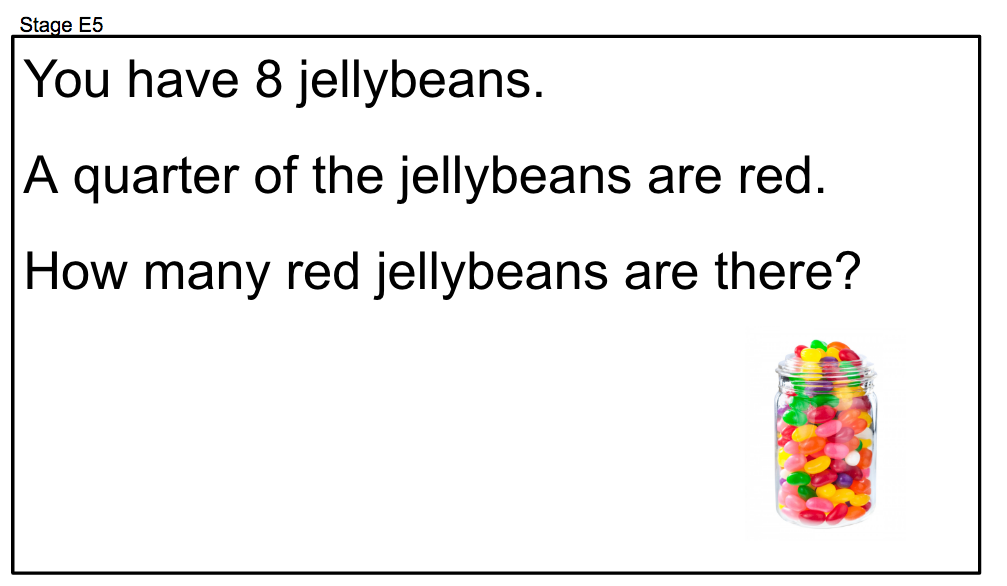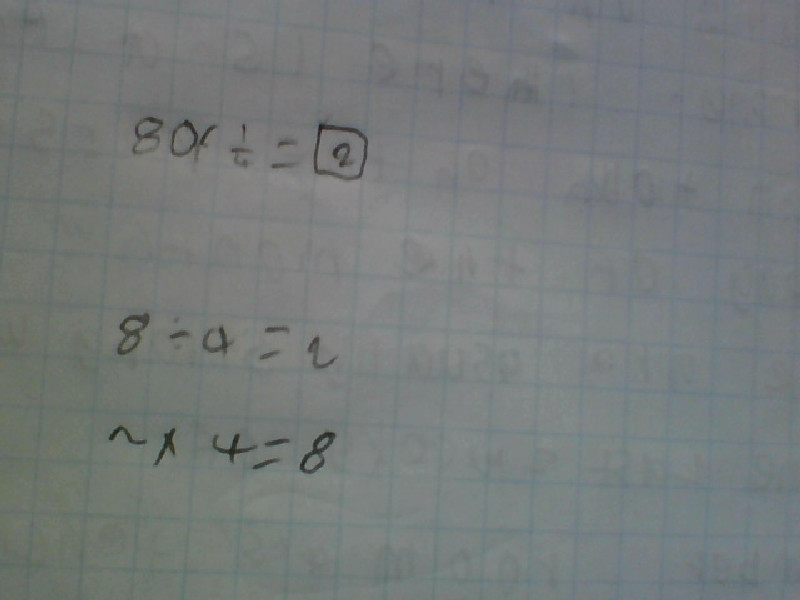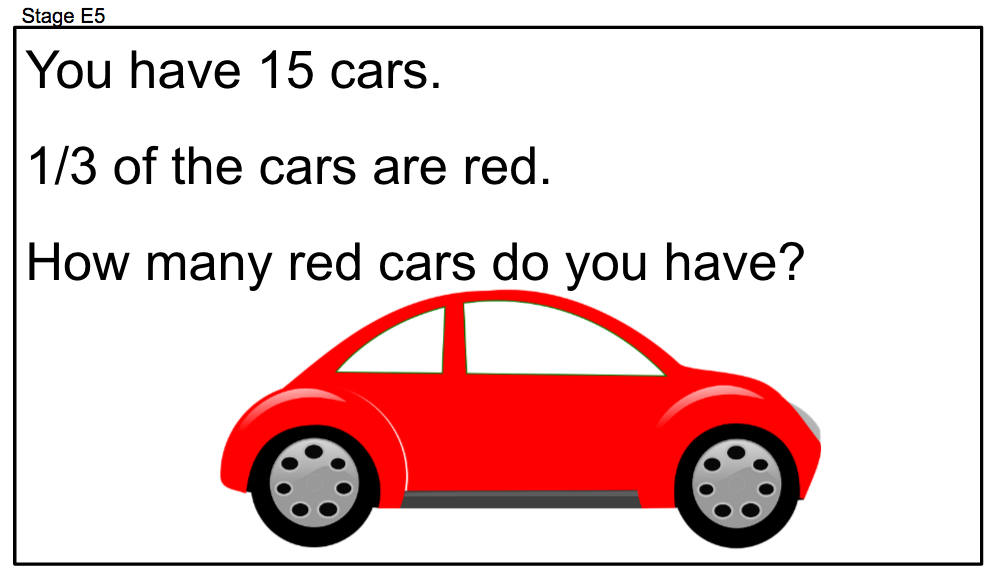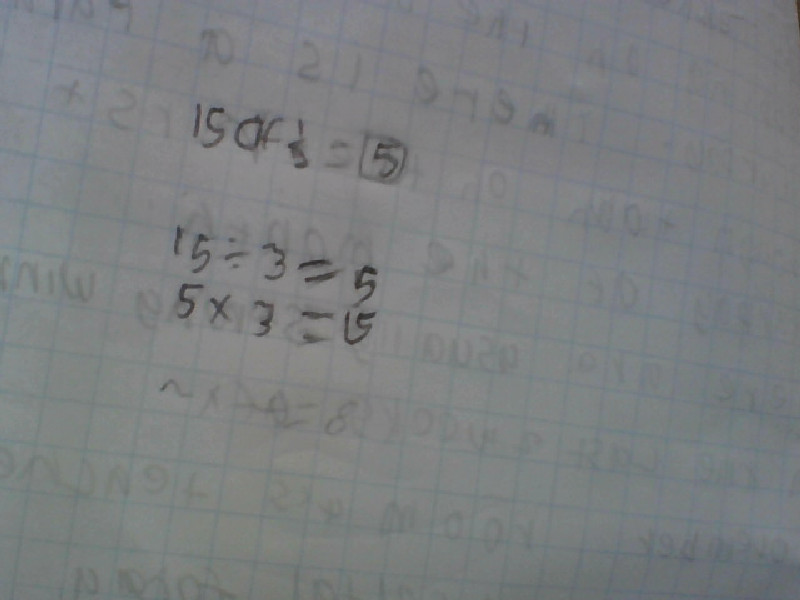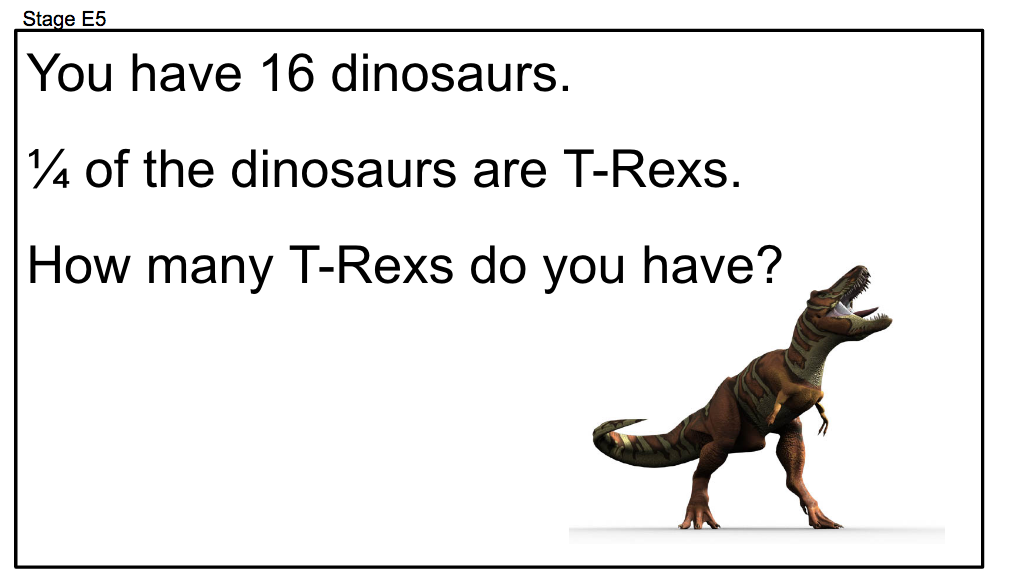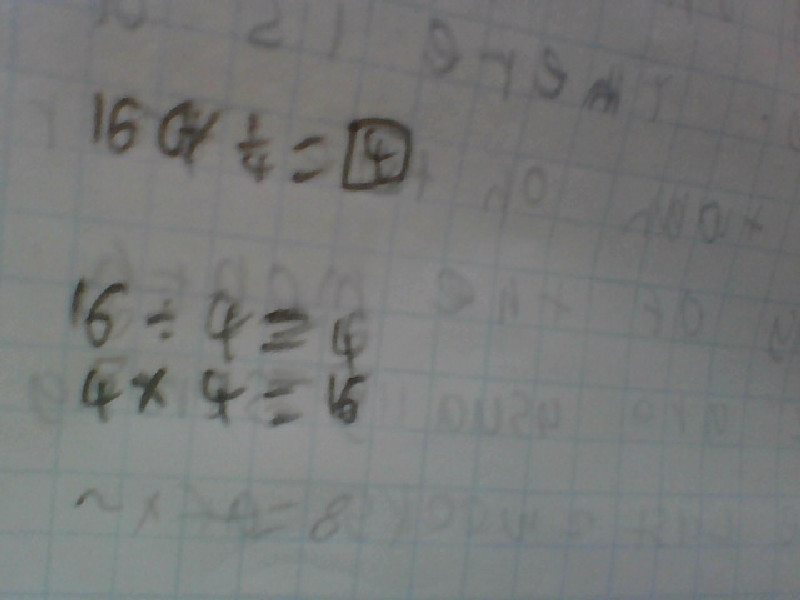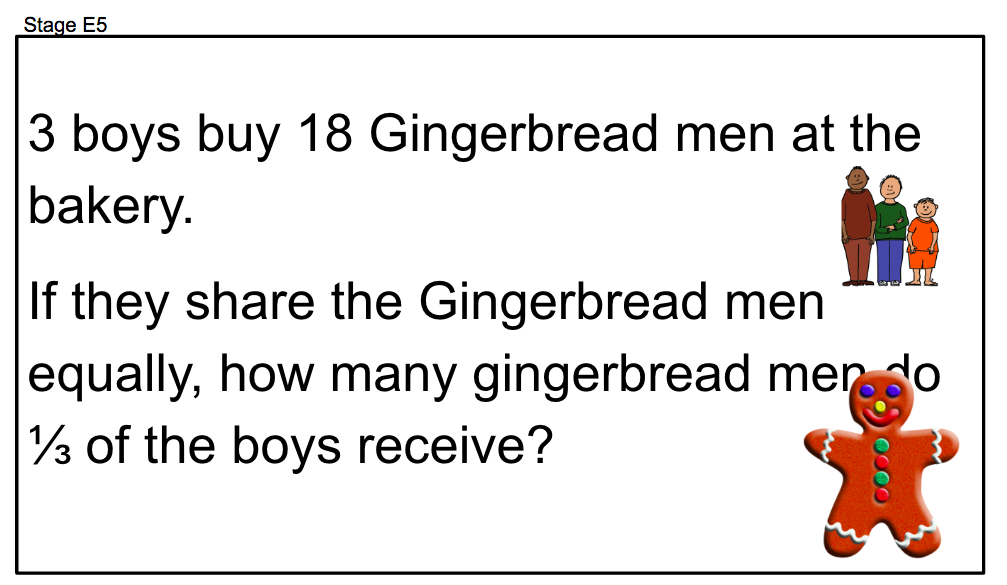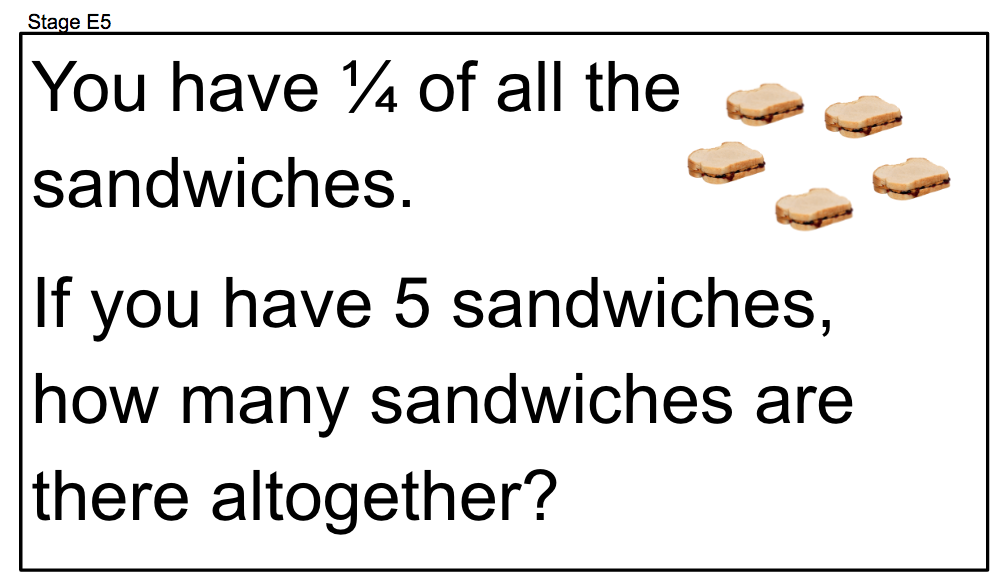¼ of 5 =20 So 4 divided by 5 =20 And 4x5=20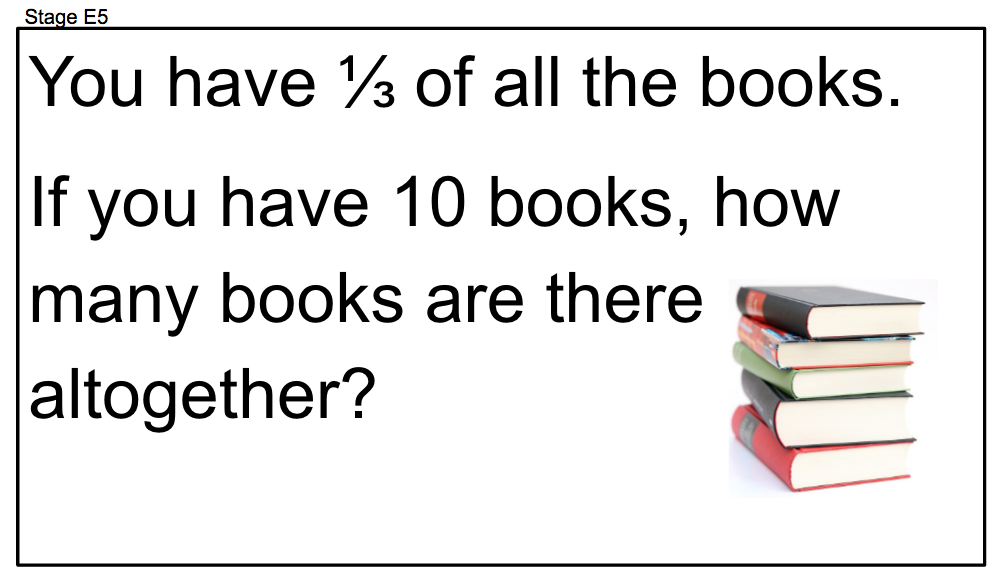⅓ of 10=30 3 divided by 10= 30 3 x 10=30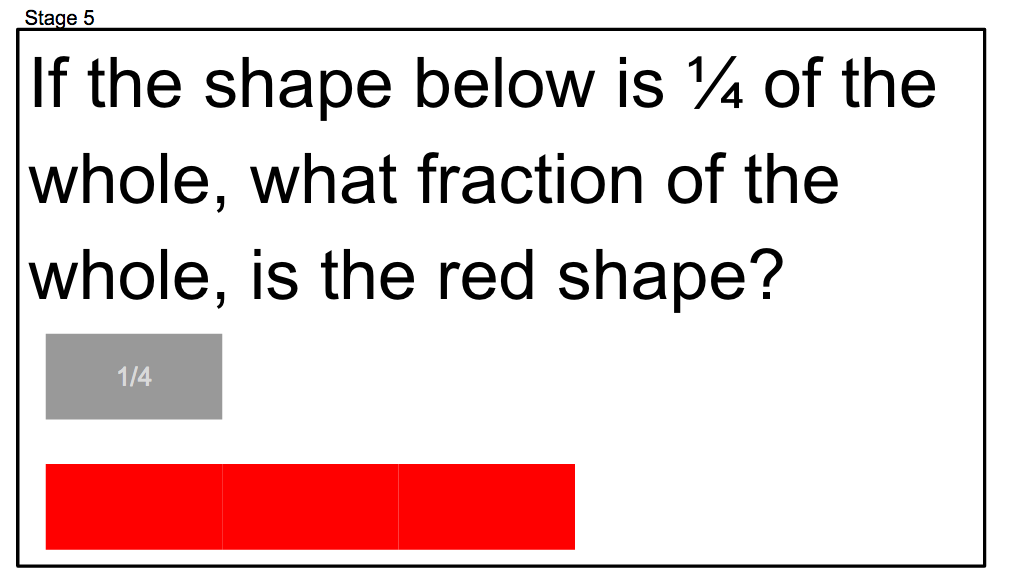¼  of 1 =3/4 ¼ of a whole = 3/4A whole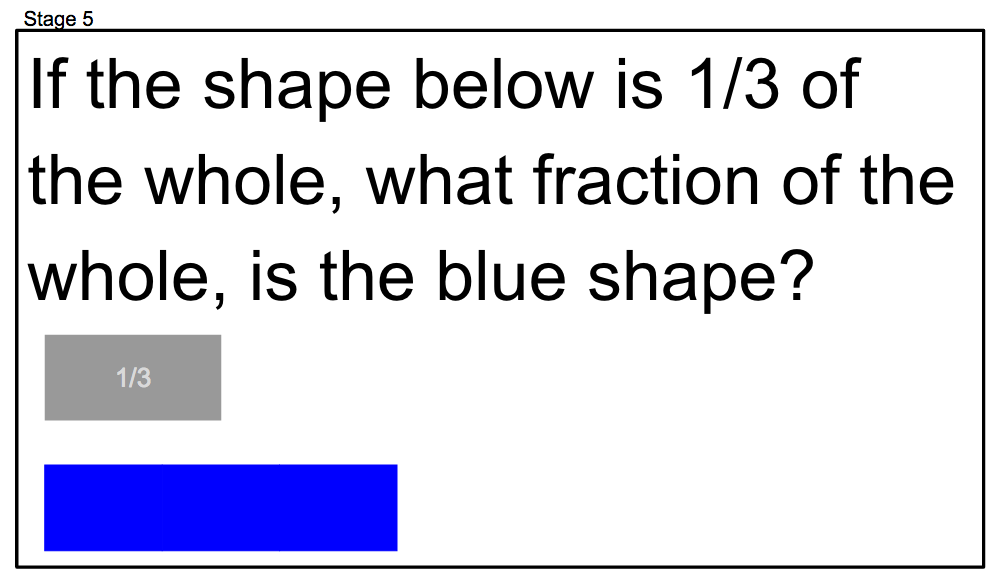⅓ of a whole = 2/3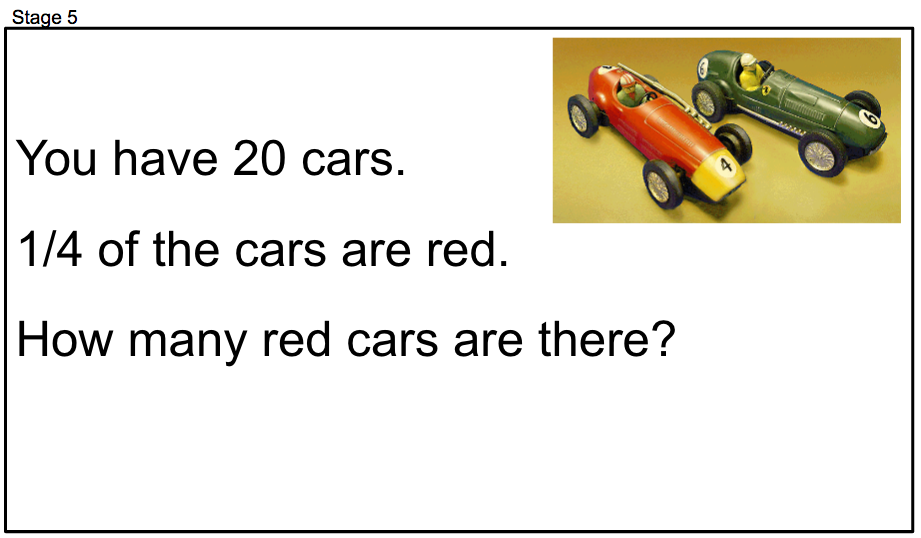20 of ¼=5 4 divided by 20=5 4 x 5 =20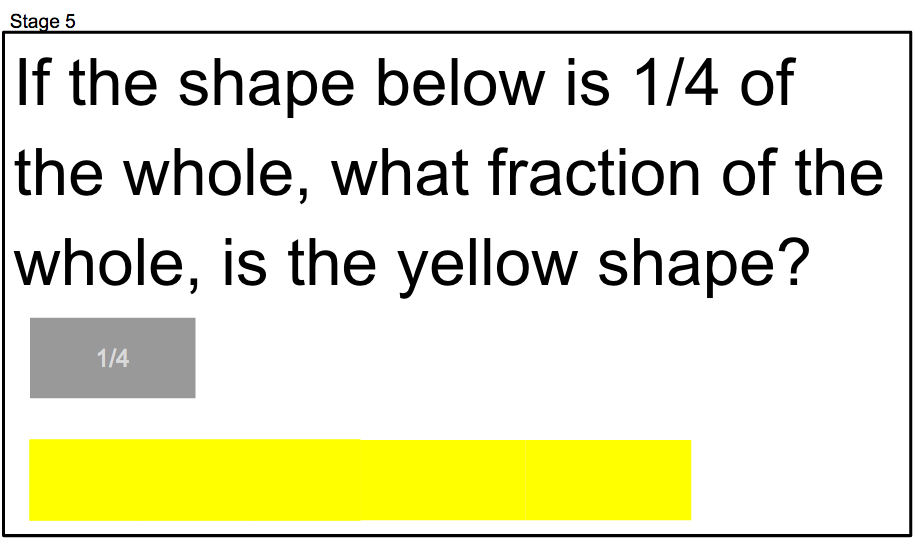¼ of a whole =3/410 of ⅓ =30 3 x 10=30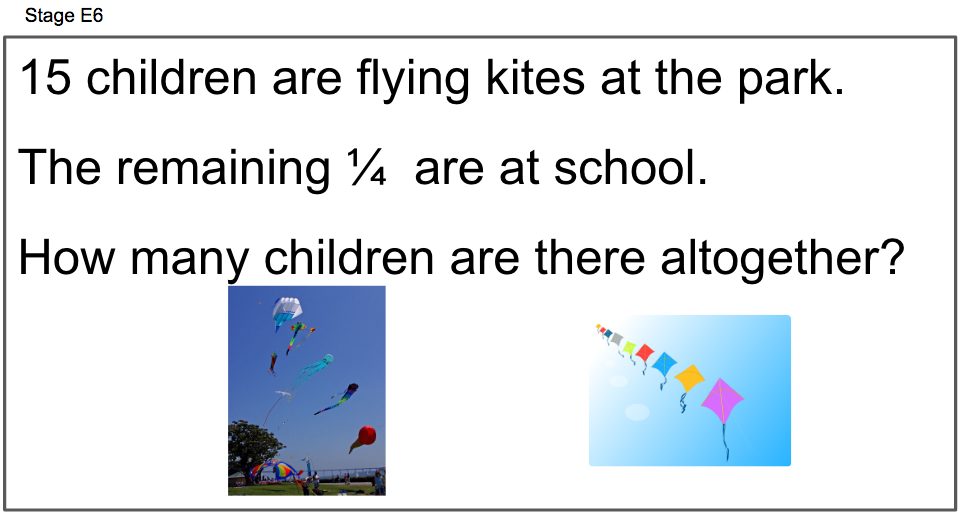15 of ¼ =60 15 x 4=60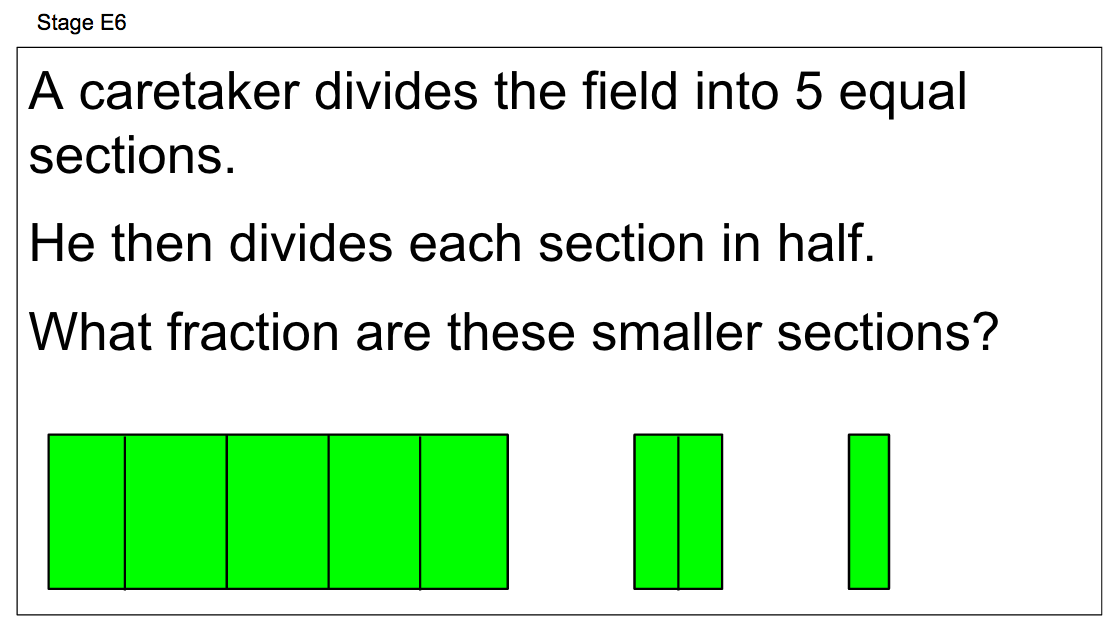2.5